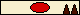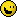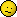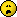# Help with maths

Other Help — Page 
Mate de Vita
Kelli2008 Oct 4 • 2453
159 ₧
2 (unrelated) inequalities I have to solve:

sqrt(1-x) - sqrt(x) > 3/sqrt(5)

and

sqrt(12-(12/x^2)) - sqrt(x^2-(12/x^2)) < x^2

I can't figure out what to do with the square roots. I tried multiplying the first with (sqrt(1-x) + sqrt(x)) but that just moves the square roots to the other side. How do I begin solving an inequality like this?
...and that's the bottom line because Mate de Vita said so.
 ≡ 2011 Nov 24 at 10:58 PST — Ed. 2011 Nov 24 at 10:59 PSTDown Rodeo
Cap'n Moth of the Firehouse2007 Oct 19 • 5486
57,583 ₧
OK, so, my first question is what are these for? If they're for a homework question or such I am slightly confused as Wolfram Alpha says there are no solutions to the first one. I'm inclined to agree. Certainly there's no way to make it much nicer.

Second one: Wolfie. It's a bit complicated. 4th roots of 3? No thanks.
 ≡ 2011 Nov 24 at 14:44 PSTRockbomb
Dog fucker (but in a good way now)2009 Nov 13 • 2045
I have no idea what's going on, but I'm gonna just agree with DR in attempt to make myself appear intelligent. I hope you're right, DR... my reputation depends on it.
 ≡ 2011 Nov 24 at 15:26 PSTSRAW
Rocket Man2007 Nov 6 • 2525
601 ₧
err can't you just solve the first one by using a calculator on the right side and factorizing on the left then send just the square over?
 ≡ 2011 Nov 24 at 18:32 PSTSRAW
Rocket Man2007 Nov 6 • 2525
601 ₧
and also on the left side, doesnt sqrt(1-x) = sqrt(1) - sqrt(x), in which case it should be easierbut I'm probably wrong≡ 2011 Nov 24 at 18:34 PSTMate de Vita
Kelli2008 Oct 4 • 2453
159 ₧
Down Rodeo said:
OK, so, my first question is what are these for? If they're for a homework question or such I am slightly confused as Wolfram Alpha says there are no solutions to the first one. I'm inclined to agree. Certainly there's no way to make it much nicer.

Second one: Wolfie. It's a bit complicated. 4th roots of 3? No thanks.

Yeah, the first one is a bit weird, since the roots of x and 1-x would imply that x is between 0 and 1 (otherwise the roots wouldn't be real numbers), which would mean those two square roots would also be between 0 and 1. I don't see how you could subtract two numbers like that to get something greater than 3/sqrt(5) (which is greater than 1).

This was kind of homework, it was a preparation for a test. Hopefully I don't get something like this in the actual test...and that's the bottom line because Mate de Vita said so.
 ≡ 2011 Nov 25 at 01:30 PSTDown Rodeo
Cap'n Moth of the Firehouse2007 Oct 19 • 5486
57,583 ₧
Just ask the teacher. As far as I can tell there is no solution to the first one. Moreover I can see no situation where you'd have to manipulate something like this, be it in further education or a job or in day-to-day life.
 ≡ 2011 Nov 25 at 05:49 PSTPage